﻿ 强震作用下高填方边坡变形与稳定性研究 Study on Deformation and Stability of High Filled Slopes under Strong Earthquakes

Vol. 11  No. 03 ( 2021 ), Article ID: 41180 , 18 pages
10.12677/AG.2021.113027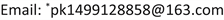Study on Deformation and Stability of High Filled Slopes under Strong Earthquakes

Xinjie Zhao, Chunqing Xie, Kai Pan*, Hang Li

Guangdong China Coal Jiangnan Engineering Survey and Design Co. Ltd., Guangzhou GuangdongReceived: Feb. 20th, 2021; accepted: Mar. 17th, 2021; published: Mar. 24th, 2021ABSTRACT

Foundation stability under strong earthquakes is one of the key engineering geological issues that restrict construction in high seismic intensity areas. Based on the site seismic conditions, based on the results of the seismic hazard probability analysis, artificially synthesize seismic waves, and establish a finite element numerical calculation model, input artificially synthesized seismic waves, and apply different fill heights, different seismic intensities, and different site conditions to high fill slope The dynamic response under different grading ratios is analyzed, relying on the huge high filling project of the western plateau airport. Results show that the response of the top slope of the fill slope is the most severe, the second is the foot of the slope, the middle is the weakest, and the displacement and acceleration have significant elevation amplification effects. The fill slope has typical characteristics of late instability and failure, and is closely related to groundwater engineering effects, under earthquake. The research results can provide references for similar engineering design, construction, scientific research, disaster prevention and mitigation in areas with high earthquake intensity.

Keywords:Severe Strong Earthquake, High Seismic Intensity, Foundation Stability, Giant High Fill Slope, Ground Motion Response, Groundwater Engineering Effect, Disaster Prevention and Mitigation1. 引言

2. 高填方边坡的主要震害

2.1. 填方体竖向不均匀沉陷

2.2. 填方边坡开裂、错落、垮塌、滑移

2.3. 填方顶面的隆起、拉裂

2.4. 支挡结构和防护工程的震害

3. 高填方边坡地震动响应特征分析

3.1. 工程概况Figure 1. Engineering features of ganzi airport in construction

1) 区域地质情况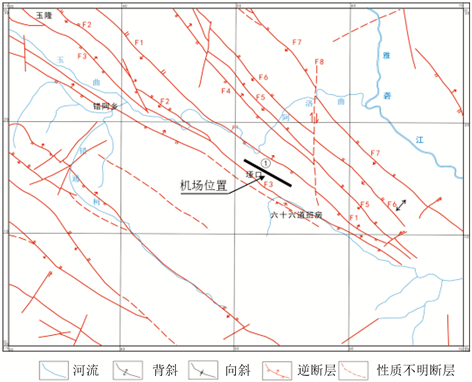Figure 2. Geological Structure Outline of Ganzi Airport

2) 工程区地形地貌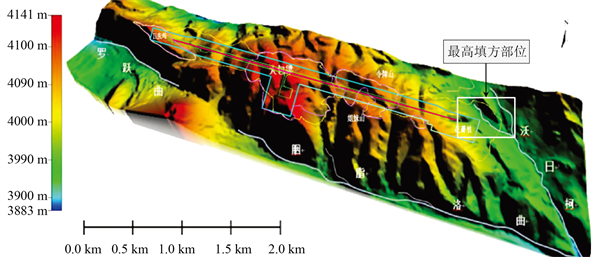Figure 3. Terrain and geomorphic features of the engineering area

3) 工程区岩土特性

3.2. 边坡动力响应时程分析

1) 计算模型的建立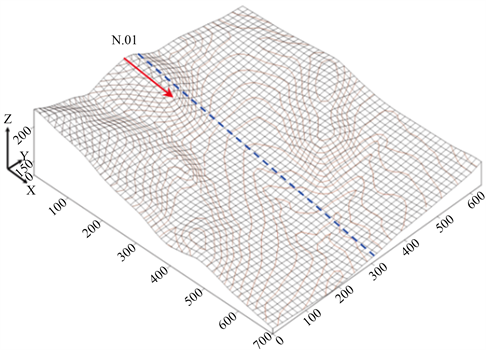Figure 4. Overall distribution characteristics before filling in the study area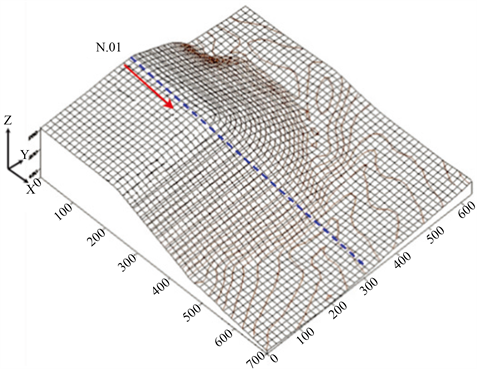Figure 5. Overall distribution characteristics after filling in the study area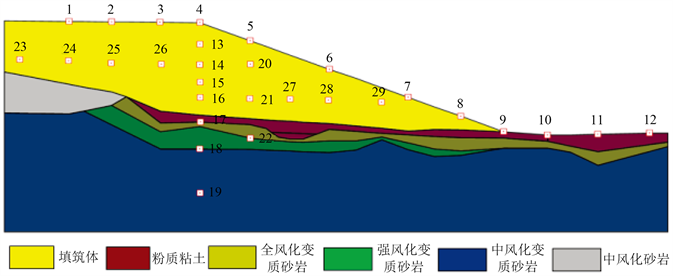Figure 6. Computational model of rock and soil layeringTable 1. Physical and mechanical parameters of calculation model

2) 地震波的合成

$a\left(t\right)=f\left(t\right)\underset{k=0}{\overset{n}{\sum }}{A}_{k}\mathrm{cos}\left({\omega }_{k}t+{\psi }_{k}\right)$ (式1)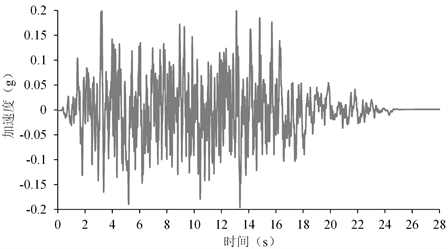Figure 7. 10% acceleration time history curve

3) 边界条件设定

① 底部边界假定水平和竖直方向均为固定；② 侧向边界由于斜坡体存在自重应力，且自重应力对填方体的固结沉降和斜坡稳定性影响非常大，地震作用下基岩及填方体向后缘无限延伸，水平位移较小，因此假定水平向固定，竖直向自由；③ 顶部边界水平和竖直方向均为自由；④ 阻尼比系数λ = 0.05；⑤ 放坡比1:2.5。

4) 时程计算方案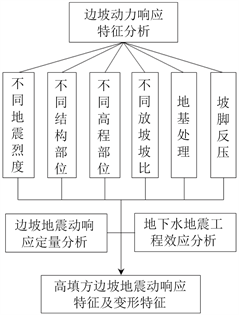Figure 8. Ground motion analysis technology route

5) 结果分析

① 地震烈度对边坡变形的影响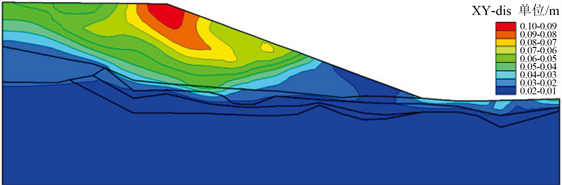Figure 9. Characteristics of permanent deformation of slope with earthquake intensity of 6.5 degrees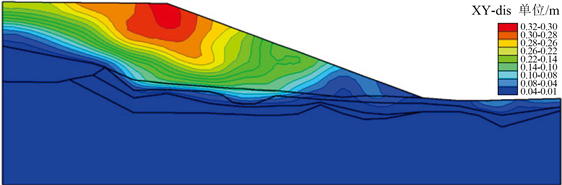Figure 10. Characteristics of permanent deformation of slopes with earthquake intensity of 8.0 degrees

② 边坡不同坡比动力响应分析

A——坡比1:2.5放坡与1:3.0不进行地基处理条件下动力响应对比分析；

B——坡比1:2.5条件下地基处理前后边坡动力响应分析；

C——坡比1:2.5放坡与1:3.0地基处理后动力响应对比分析。

(A) 坡比1:2.5与1:3.0不进行地基处理条件下动力响应对比分析

(B) 坡比1:2.5地基处理前后边坡动力响应分析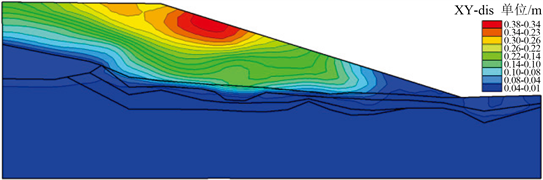Figure 11. Intensity 8.0 Permanent deformation of slope (1:3.0 Gradation)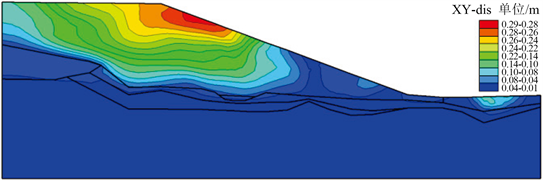Figure 12. Characteristics of permanent deformation of slope after foundation treatment (1:3.0 Gradation)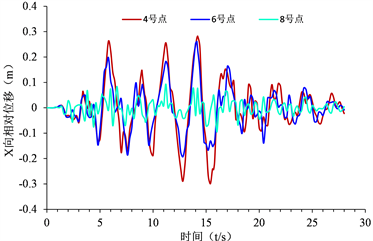Figure 13. X-direction relative displacement of slope monitoring point before replacement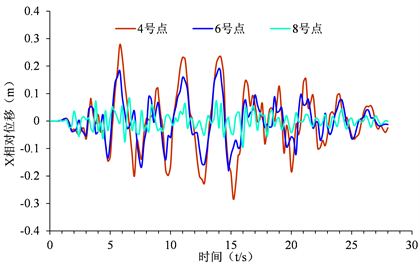Figure 14. Relative displacement in X direction of slope monitoring point after replacement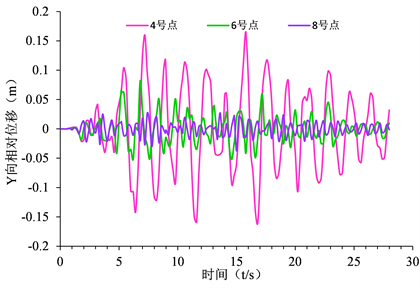Figure 15. Relative displacement in Y direction of monitoring point of slope surface before replacement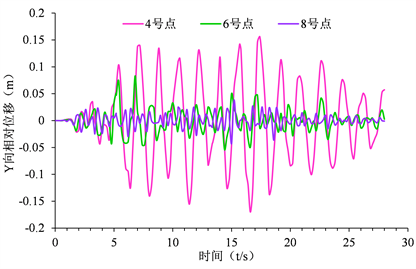Figure 16. Relative displacement in Y direction of slope monitoring point after replacement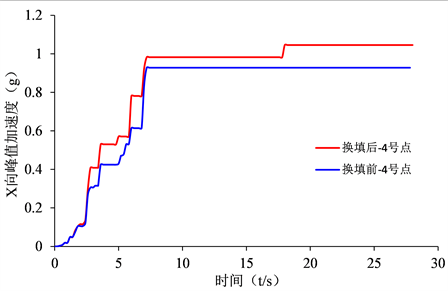Figure 17. 4# point X-direction peak acceleration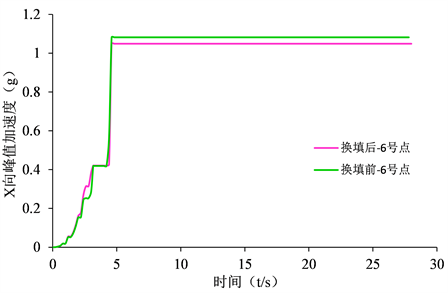Figure 18. 4# point Y-direction peak acceleration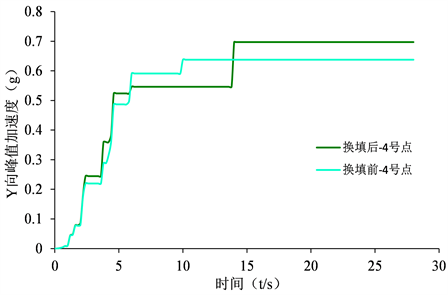Figure 19. 6# point X-direction peak acceleration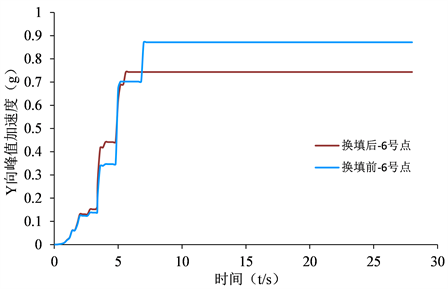Figure 20. 6# point Y-direction peak acceleration

(C) 坡比1:2.5与1:3.0地基处理后边坡动力响应分析

1:2.5放坡地基处理后坡顶4号监测点X向最大相对位移0.278 m，Y向最大相对位移0.156 m，边坡永久变形量0.289 m；1:3.0放坡地基处理后X向最大相对位移0.266 m，Y向最大相对位移0.178 m，边坡永久变形量0.292 m。初步得出，增大放坡坡比后结合进行地基处理对减小边坡X向永久位移有效，对减小Y向永久作用不明显。6号、8号监测点显示增大坡比并进行地基处理对减小边坡Y向永久位移具有一定效果，但对减小X向永久位移效果不明显。

③ 坡脚反压对边坡动力响应的影响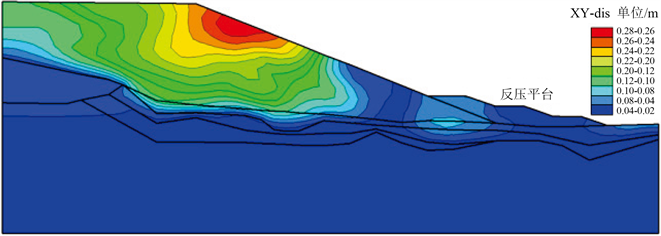Figure 21. 1:3.0 Characteristics of permanent deformation of slope foot anti-pressure slopeTable 2. Changes in peak acceleration at slope monitoring points before and after backpressure

④ 边坡的位移及加速度高程放大效应特征Table 3. Statistics of ground motion response data at vertical monitoring points on slope tops

${H}_{dx}=0.0496{x}^{0.3901}$ (2)

${S}_{dy}=0.026{x}^{0.4244}$ (3)

X向加速度与垂向填方高度的关系函数：

${A}_{x}=9e-0.5{x}^{2}-0.0052x+0.5158$ (4)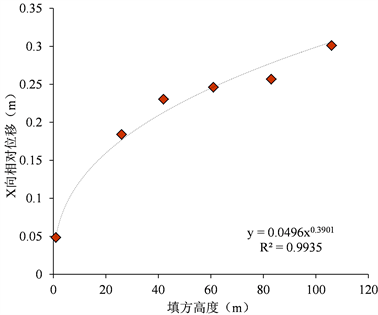Figure 22. Relation curve between vertical fill height and X relative displacement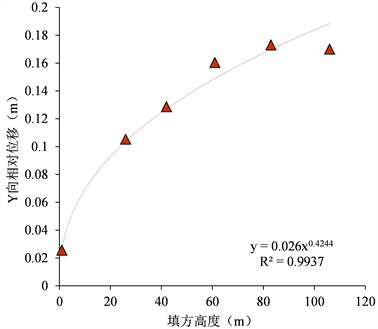Figure 23. Relation curve between vertical fill height and Y relative displacementTable 4. Data statistics of calculation results of ground motions on slope surface monitoring points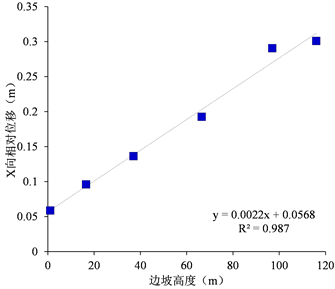Figure 24. Relationship between slope height and relative displacement in X direction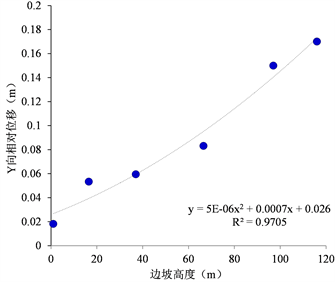Figure 25. Relationship between slope height and relative displacement in Y direction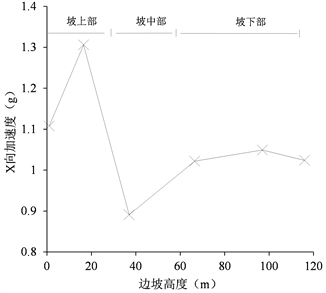Figure 26. Relationship between slope height and X-direction acceleration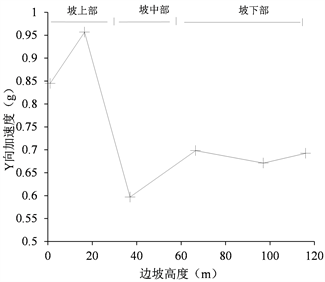Figure 27. Relationship between slope height and Y-direction acceleration

${H}_{fx}=0.0022x+0.0568$ (5)

X向加速度与边坡高度的关系函数：

${S}_{fx}=5e-06{x}^{2}+0.0007x+0.026$ (6)

4. 震后失稳机制与地下水工程效应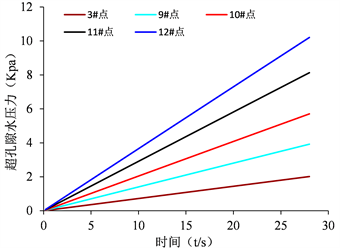Figure 28. Variation curve of super-pore water pressure under earthquake

5. 结论

1) 高地震烈度区高填方边坡地震动响应特征：① 边坡中上部地震动效应大于中下部地震动响应，坡面上部1/3至坡顶的地震动响应最剧烈；② 顶部表现出越靠近临空面地震动越强烈，向后缘延伸地震动响应逐步减弱；③ 坡体内部地震效应较弱，越靠近表面地震动响应越强烈，建设中应进行重点加强抗震设计和边坡加固工作。

2) 地基不均会产生地震波的差异性放大作用，导致坡体沉降、开裂、滑塌破坏，加剧震害。建议在填方过程中应对不均匀地基进行处理，特别是边坡部位，应重点处理以确保边坡的稳定。

3) 地震后边坡土石松动开裂，为雨水的入渗创造了条件，从而导致边坡内部地下水径流路径发生变化，降低岩土体力学强度，加速地下水渗流潜蚀，易造成震后失稳，震后应及时采取有效应的应急处置措施。

4) 地下水具有浸泡软化填方地基土，劣化岩土物理力学性能，渗流潜蚀破坏填筑体的工程效应，并且强震作用产生的高孔隙水压力和超孔隙水压力将进一步加剧边坡的变形、破坏。

Study on Deformation and Stability of High Filled Slopes under Strong Earthquakes[J]. 地球科学前沿, 2021, 11(03): 305-322. https://doi.org/10.12677/AG.2021.113027

1. 1. 谢春庆, 刘汉超. 西南地区机场建设中的主要工程地质问题[J]. 地质灾害与环境保护, 2001, 12(2): 32-35.

2. 2. 黄润秋. 汶川地震地质灾害研究[M]. 北京: 科学出版社, 2009.

3. 3. 阮立冬. 汶川大地震梁桥震害分析及梁桥抗震措施研究[J]. 科技信息, 2010(3): 321-322.

4. 4. 郑建军, 廖永石, 杜雷, 等. 青海玉树地震民用建筑震害调查和分析[J]. 建筑结构, 2013, 43(S1): 1051-1054.

5. 5. 杨理臣, 卢宁, 樊光洁, 郭鹏. 青海玉树7.1级地震灾后重建地区房屋地震易损性研究[J]. 地震工程学报, 2016, 38(S2): 314-318.

6. 6. 兰恒星, 周成虎, 高星, 等. 四川雅安芦山地震灾区次生地质灾害评估及对策建议[J]. 地理科学进展, 2013, 32(4): 499-504.

7. 7. 徐明明. 填方路堤地震响应分析[D]: [硕士学位论文]. 重庆: 重庆大学, 2009.

8. 8. Idriss, I.M. and Seed, H.B. (1968) Seismic Response of Horizontal Soil Layers. Journal of the Soil Mechanics and Foundations Division, 94, 1003-1031. https://doi.org/10.1061/JSFEAQ.0001163

9. 9. 石乃鑫. 含软弱夹层岩质边坡的地震时程响应分析[D]: [硕士学位论文]. 兰州: 兰州理工大学, 2010.

10. 10. 陈昌凯. 地震作用下边坡的有限元动力模拟[D]: [硕士学位论文]. 昆明: 昆明理工大学, 2006.

11. 11. 谭儒蛟, 李明生, 徐鹏逍, 等. 地震作用下边坡岩体动力稳定性数值模拟[J]. 岩石力学与工程学报, 2009, 28(2): 3986-3992.

12. 12. 梁力, 王伟, 李明. 某露天矿岩质高边坡地震动力响应及稳定性分析[J]. 金属矿山, 2008(8): 21-25.

13. 13. 四川赛思特科技有限责任公司. 四川甘孜格萨尔机场工程场地地震安全性评价报告[R]. 成都: 四川赛思特科技有限责任公司, 2013.

14. 14. 成都军区空军勘察设计院. 四川甘孜格萨尔机场岩土工程详细勘察报告[R]. 成都: 成都军区空军勘察设计院, 2013.

15. 15. 中华人民共和国国家质量监督检验检疫总局: GB17741-2005 [S]. 北京: 中国建筑工业出版社, 2005.

16. 16. 胡聿贤. 地震安全性评价技术教程[M]. 第4版. 北京: 地震出版社, 2011.

17. 17. 祁生文, 伍法权, 刘春玲, 等. 地震边坡稳定性的工程地质分析[J]. 岩石力学与工程学报, 2004, 23(16): 2792-2797.

18. NOTES

*通讯作者。